Four wires of equal length and of resistances 10 ohms each are connected in the form of a square. The equivalent resistance between two opposite corners of the square is :

(1) 10 ohm

(2) 40 ohm

(3) 20 ohm

(4) 10/4 ohm

Concept Questions :-

Combination of resistors
High Yielding Test Series + Question Bank - NEET 2020

Difficulty Level:

Two resistors are connected (a) in series (b) in parallel. The equivalent resistance in the two cases is 9 ohm and 2 ohms respectively. Then the resistances of the component resistors are :

(1) 2 ohm and 7 ohm

(2) 3 ohm and 6 ohm

(3) 3 ohm and 9 ohm

(4) 5 ohm and 4 ohm

Concept Questions :-

Combination of resistors
High Yielding Test Series + Question Bank - NEET 2020

Difficulty Level:

Resistors of 1, 2, 3 ohm are connected in the form of a triangle. If a 1.5-volt cell of negligible internal resistance is connected across a 3-ohm resistor, the current flowing through this resistance will be :

(1) 0.25 amp

(2) 0.5 amp

(3) 1.0 amp

(4) 1.5 amp

Concept Questions :-

Combination of resistors
High Yielding Test Series + Question Bank - NEET 2020

Difficulty Level:

Resistances of 6 ohm each are connected in the manner shown in adjoining figure. With the current 0.5 ampere as shown in figure, the potential difference ${V}_{P}-{V}_{Q}$ is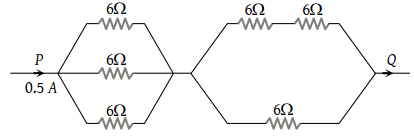(1) 3.6 V

(2) 6.0 V

(3) 3.0 V

(4) 7.2 V

Concept Questions :-

Kirchoff's voltage law
High Yielding Test Series + Question Bank - NEET 2020

Difficulty Level:

The equivalent resistance of the arrangement of resistances shown in adjoining figure between the points A and B is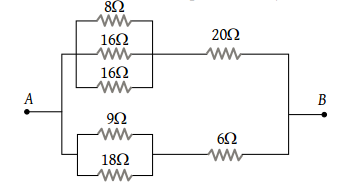(1) 6 ohm

(2) 8 ohm

(3) 16 ohm

(4) 24 ohm

Concept Questions :-

Combination of resistors
High Yielding Test Series + Question Bank - NEET 2020

Difficulty Level:

In the network of resistors shown in the adjoining figure, the equivalent resistance between A and B is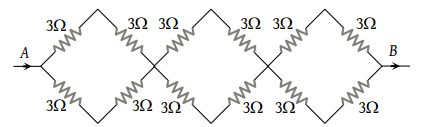(1) 54 ohm

(2) 18 ohm

(3) 36 ohm

(4) 9 ohm

High Yielding Test Series + Question Bank - NEET 2020

Difficulty Level:

A wire is broken in four equal parts. A packet is formed by keeping the four wires together. The resistance of the packet in comparison to the resistance of the wire will be

(1) Equal

(2) One fourth

(3) One eight

(4) $\frac{1}{16}th$

High Yielding Test Series + Question Bank - NEET 2020

Difficulty Level:

Four resistances are connected in a circuit in the given figure. The electric current flowing through 4 ohm and 6 ohm resistance is respectively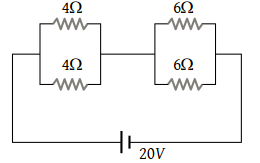(1) 2 amp and 4 amp

(2) 1 amp and 2 amp

(3) 1 amp and 1 amp

(4) 2 amp and 2 amp

High Yielding Test Series + Question Bank - NEET 2020

Difficulty Level:

An infinite sequence of resistance is shown in the figure. The resultant resistance between A and B will be, when R1 = 1 ohm and R2 = 2 ohm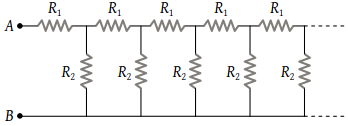(1) Infinity

(2) 1 Ω

(3) 2 Ω

(4) 1.5 Ω

High Yielding Test Series + Question Bank - NEET 2020

Difficulty Level:

In the figure, the value of resistors to be connected between C and D so that the resistance of the entire circuit between A and B does not change with the number of elementary sets used is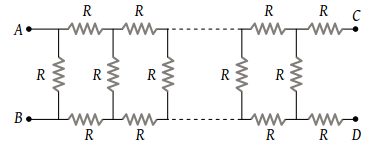(1) R

(2) $R\left(\sqrt{3}-1\right)$

(3) 3 R

(4) $R\left(\sqrt{3}+1\right)$

Concept Questions :-

Combination of resistors